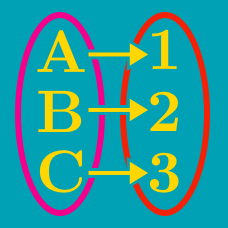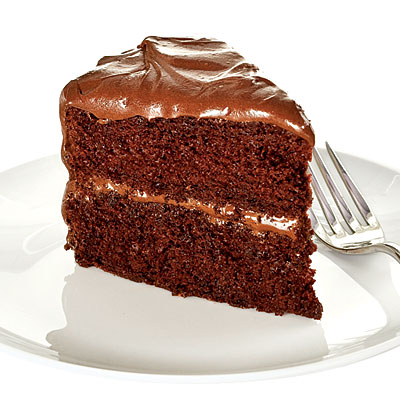Probability

# Bijections: Level 5 Challenges

$\large\sum _{ i=1 }^{ n }{ \left\lfloor \frac { A }{ B } i \right\rfloor } +\sum _{ j=1 }^{ m }{ \left\lfloor \frac { B }{ A } j \right\rfloor } = f(n)f(m)$

Let $$A,B$$ be odd natural numbers that are relatively prime, and let $$n= \frac { B-1 }{ 2 }$$, $$m=\frac { A-1 }{ 2 }$$ such that the equation above is fulfilled. If $$f$$ is a linear function with $$f(1) > 0$$, what is the sum of the coefficients of $$f(x)$$?

All 20 diagonals are drawn in a regular octagon. At how many distinct points in the interior of the octagon (not on the boundary) do two or more diagonals intersect?

Details and assumptions

A regular octagon has $${ 8 \choose 2} - 8 = 28 - 8 = 20$$ diagonals.

How many permutations $$\sigma$$ of the set $$\{1, 2, \ldots, 15\}$$ are there such that $$\sigma (1) = 1, \lvert \sigma (n) - \sigma (n-1) \rvert \leq 2$$ for $$2 \leq n \leq 15$$?

Details and assumptions

$$\sigma(n)$$ denotes the $$n^{th}$$ position of the permutation.

Brilli the ant is considering the complexities of a 5-dimensional universe. She wants to count the number of integer points that are distance $$\sqrt{n}$$ away from the origin. Let $$T_n$$ be the set of ordered 5-tuples of integers $$(a_1,a_2,a_3,a_4,a_5)$$ such that $$a_1^2 + a_2^2 + a_3^2 + a_4^2 + a_5^2 = n.$$ However, being an ant, she doesn't have enough toes to record numbers that are above 10. Let $$D_n$$ be the units digit of $$\vert T_n \vert.$$

Determine $$\sum_{i = 1}^{5678} D_i.$$

Details and assumptions

Note: You are not asked to find the last digit of the sum, but the sum of all the last digits.

For a set $$S$$, $$| S |$$ denotes the number of elements in $$S$$. You can read up on set notation.A man is hired to cut cake at a party. He was informed the day before the party that $$28$$ people could definitely attend the party but one person wasn't sure if he could make it or not. To make sure everything would be fair, he decided the cut the circular cake into slices such that if either $$28$$ or $$29$$ people attended the party, the cake could be distributed evenly to all attendees. At first he thought he needed $$28*29=812$$ slices but his wife informed him that he was being an idiot.

What is the minimum number of slices the cake cutter needs to slice the cake into such that either $$28$$ or $$29$$ people can attend the party and the cake can still be distributed evenly?

×